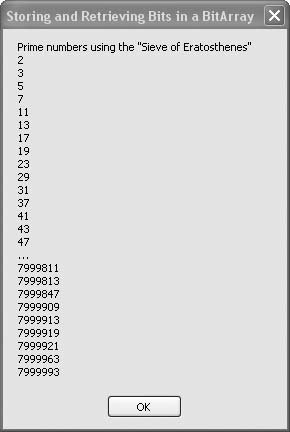# Recipe 6.25. Storing and Retrieving Bits in a BitArray

#### Problem

You want to store and retrieve a lot of bits without wasting memory and without sacrificing speed of operation.

#### Solution

Sample code folder: Chapter 06\GetPrimes

Use a BitArray to store and access individual bits in memory efficiently.

#### Discussion

The BitArray object lets you access bits by indexed position, and all the details of decoding which bit position of which byte the bit is stored in are taken care of transparently behind the scenes. A BitArray of 80 bits is actually stored in 10 bytes of memory.

To demonstrate using a BitArray, we've created a module named Eratosthenes.vb that contains code to find all prime numbers between 2 and 8,000,000 very quickly. The 8 million bits are stored in 1 million bytes of memory, and the individual bits are accessed using indexes in the range 0 to 8,000,000.

The Sieve of Eratosthenes (http://en.wikipedia.org/wiki/Sieve_of_Eratosthenes) works by first setting all bits to 1, or TRue. The BitArray can be instantiated with a count and an optional second Boolean parameter that presets all bits to true or False. In this case, true sets them all to 1. Starting with 2, each prime number, or bit that is set, clears all bits that are exact multiples of that number. So, for instance, bit 2 is kept at TRue, but bits 4, 6, 8, and so on, are all set to False. This marks all even numbers except for 2 as nonprime. Similarly, bit 3 is left TRue, and bits 6, 9, 12, 15, etc., are set to False to mark all multiples of 3 as nonprime. This looping technique very quickly sets all bits in the BitArray that appear in prime number positions to True and all other bits to False.

The Eratosthenes module contains the BitArray itself, a Sieve() method to set all the prime number bits as described earlier, and a GetBit() function to retrieve the bit at any location, converting the bit's true or False Boolean value to a 1 or 0 integer value:

` Module Eratosthenes    Private Const MaxNumber As Integer = 8000000    Private PrimeStorage As New BitArray(MaxNumber, True)    Public Sub Sieve()       ' ----- Get all the prime numbers from 1 to MaxNumber.       Dim index As Integer = 1       Dim counter As Integer       ' ----- Scan through all primes.       Do While (index < (MaxNumber - 1))          index += 1          If (PrimeStorage(index) = True) Then             ' ----- Found a prime. Set all of its multiples             '       to non-prime.             For counter = index * 2 To MaxNumber - 1 _                   Step index                PrimeStorage(counter) = False             Next counter          End If       Loop    End Sub    Public Function GetBit(ByVal index As Integer) As Integer       ' ----- Retrieve the status of a single prime bit.       If (PrimeStorage(index) = True) Then _          Return 1 Else Return 0    End Function End Module `

The following block of code demonstrates the BitArray in action, displaying the prime numbers up to the size of the BitArray. To prevent information overload, only the first and last few numbers in the desired range are formatted into a string for display, as there are a lot of prime numbers between 0 and 8,000,000:

` Dim result As New System.Text.StringBuilder Dim counter As Integer Dim needBreak As Boolean = True result.AppendLine( _    "Prime numbers using the ""Sieve of Eratosthenes""") ' ----- Generate the primes. Sieve() ' ----- Report each prime. For counter = 2 To 7999999    If (GetBit(counter) = 1) Then       If (counter < 50) Or (counter > 7999800) Then          ' ----- Only show a limited number of primes.          result.AppendLine(counter)       ElseIf (needBreak = True) Then          ' ----- Show that we are leaving something out.          result.AppendLine("…")          needBreak = False       End If    End If Next counter MsgBox(result.ToString()) `

Figure 6-25 shows the partial list of all the prime numbers as determined by the bits in the BitArray. On your system there could be less than a second's delay during the computation and display of these prime numbers!

##### Figure 6-25. All the prime numbers between 0 and 8,000,000, calculated quickly using a BitArrayVisual Basic 2005 Cookbook: Solutions for VB 2005 Programmers (Cookbooks (OReilly))
ISBN: 0596101775
EAN: 2147483647
Year: 2006
Pages: 400

Similar book on Amazon